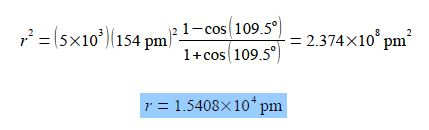# Polymer Chemistry: Mathematical Explanation

Long polymer molecules tend to coil up rather than being stretched out straight, below Figure is an example of a short polythene molecule.Randomly coined polyethylene, H= gray, C= Dark gray

Looking at a large number of such randomly coiled molecules, the average end-to-end distance r of the polymer molecules is given by

r2 = Nʃ2 [(1 – cosθ) / (1+ cosθ)]

Where the polymer consists of N chemical bonds making up the backbone of the polymer chain, with a monomer length of ʃ, and θ the C-atom bond angle. Calculate the average end-to-end distance, r, for a polymer with θ = 109.5° (the tetrahedral angle), ʃ = 154 pm and N= 5×103. Specify the units for your calculated r value. The symbol pm stands for a picometre or 10-12 m which is a common multiple unit for the sizes of atoms and chemical bonds.

Solution

r2 = Nʃ2 [(1 – cosθ) / (1+ cosθ)]

Substituting in the values of the parameters for the variables givesAverage end-to-end distance for the polymer chemistry r =1.5408×104 pm which is much less (≈ 2%) of the stretched out “linear” length of polymer, 77×104 pm. Macromolecules, both natural and synthetic, will be covered in detail in your Chemistry lectures. Also it was not necessary to convert to metres, but it was necessary to have the units of the length in the equation.